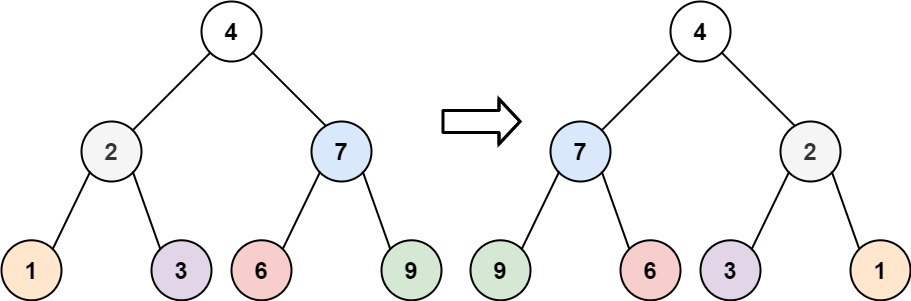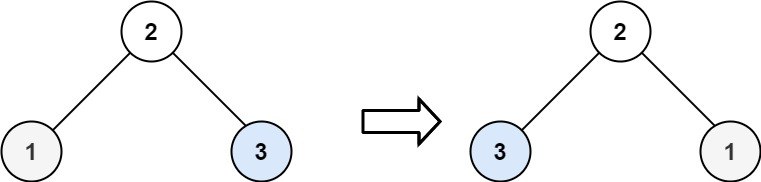# 226.invert-binary-tree

## Statement

• Difficulty: Easy
• Tag: `树` `深度优先搜索` `广度优先搜索` `二叉树```````输入：root = [4,2,7,1,3,6,9]

````````````输入：root = [2,1,3]

``````

``````输入：root = []

``````

• 树中节点数目范围在 `[0, 100]`
• `-100 <= Node.val <= 100`

• Difficulty: Easy
• Tag: `Tree` `Depth-First Search` `Breadth-First Search` `Binary Tree`

Given the `root` of a binary tree, invert the tree, and return its root.

Example 1:``````Input: root = [4,2,7,1,3,6,9]
Output: [4,7,2,9,6,3,1]
``````

Example 2:``````Input: root = [2,1,3]
Output: [2,3,1]
``````

Example 3:

``````Input: root = []
Output: []
``````

Constraints:

• The number of nodes in the tree is in the range `[0, 100]`.
• `-100 <= Node.val <= 100`

## Solution

``````# Definition for a binary tree node.
# class TreeNode:
#     def __init__(self, val=0, left=None, right=None):
#         self.val = val
#         self.left = left
#         self.right = right
class Solution:
def dfs(self, rt: TreeNode) -> None:
if not rt:
return

rt.left, rt.right = rt.right, rt.left
self.dfs(rt.left)
self.dfs(rt.right)

def invertTree(self, root: TreeNode) -> TreeNode:
self.dfs(root)
return root
``````